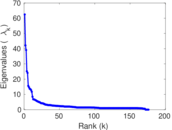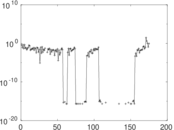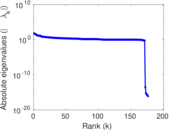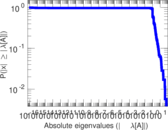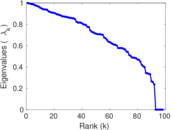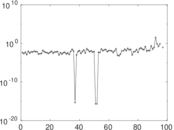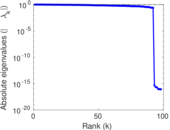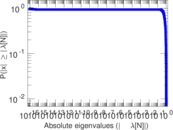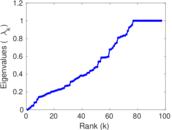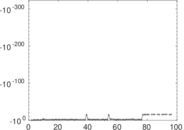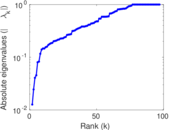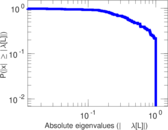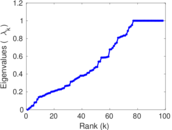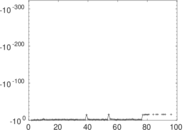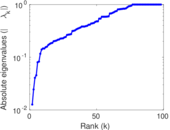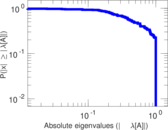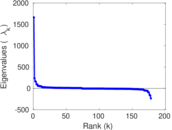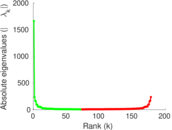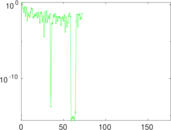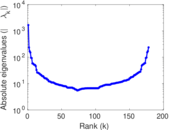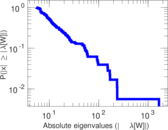# Wikibooks edits (tt)

This is the bipartite edit network of the Tatar Wikibooks. It contains users and pages from the Tatar Wikibooks, connected by edit events. Each edge represents an edit. The dataset includes the timestamp of each edit.

 Code `btt` Internal name `edit-ttwikibooks` Name Wikibooks edits (tt) Data source http://dumps.wikimedia.org/ AvailabilityDataset is available for download Consistency checkDataset passed all tests Category Authorship network Dataset timestamp 2017-10-20 Node meaning User, article Edge meaning Edit Network formatBipartite, undirected Edge typeUnweighted, multiple edges Temporal dataEdges are annotated with timestamps

## Statistics

 Size n = 1,671 Left size n1 = 178 Right size n2 = 1,493 Volume m = 2,861 Unique edge count m̿ = 1,921 Wedge count s = 203,822 Claw count z = 21,808,444 Cross count x = 1,944,560,379 Square count q = 20,276 4-Tour count T4 = 981,366 Maximum degree dmax = 807 Maximum left degree d1max = 807 Maximum right degree d2max = 42 Average degree d = 3.424 30 Average left degree d1 = 16.073 0 Average right degree d2 = 1.916 28 Fill p = 0.007 228 49 Average edge multiplicity m̃ = 1.489 33 Size of LCC N = 1,391 Diameter δ = 14 50-Percentile effective diameter δ0.5 = 3.732 08 90-Percentile effective diameter δ0.9 = 6.516 36 Median distance δM = 4 Mean distance δm = 4.586 32 Gini coefficient G = 0.645 767 Balanced inequality ratio P = 0.259 874 Left balanced inequality ratio P1 = 0.126 180 Right balanced inequality ratio P2 = 0.368 053 Relative edge distribution entropy Her = 0.785 942 Power law exponent γ = 4.491 31 Tail power law exponent γt = 2.511 00 Tail power law exponent with p γ3 = 2.511 00 p-value p = 0.000 00 Left tail power law exponent with p γ3,1 = 1.791 00 Left p-value p1 = 0.123 000 Right tail power law exponent with p γ3,2 = 5.351 00 Right p-value p2 = 0.048 000 0 Degree assortativity ρ = −0.167 158 Degree assortativity p-value pρ = 1.661 14 × 10−13 Spectral norm α = 62.440 1 Algebraic connectivity a = 0.012 672 7 Spectral separation |λ1[A] / λ2[A]| = 1.479 56 Controllability C = 1,262 Relative controllability Cr = 0.786 783

## Plots

### Fruchterman–Reingold graph drawing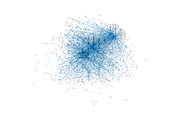### Degree distribution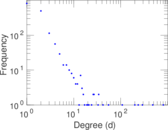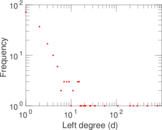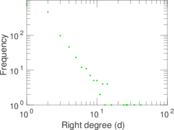### Cumulative degree distribution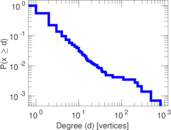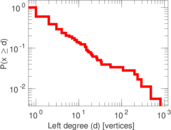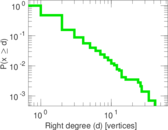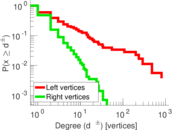### Lorenz curve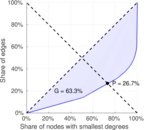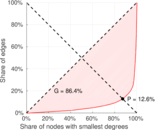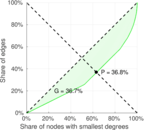### Spectral distribution of the adjacency matrix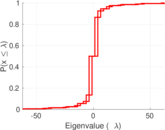### Spectral distribution of the normalized adjacency matrix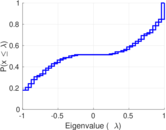### Spectral distribution of the Laplacian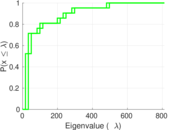### Spectral graph drawing based on the adjacency matrix### Spectral graph drawing based on the Laplacian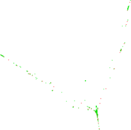### Spectral graph drawing based on the normalized adjacency matrix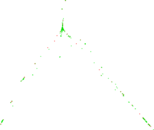### Degree assortativity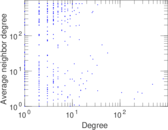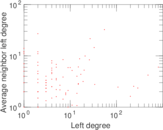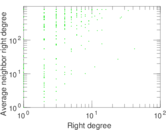### Zipf plot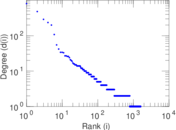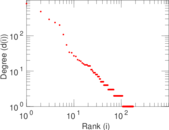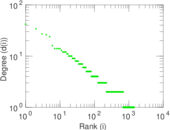### Hop distribution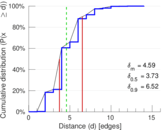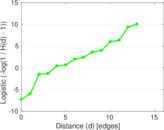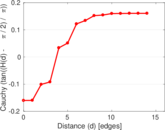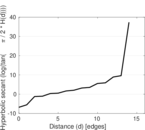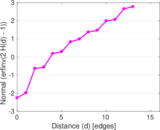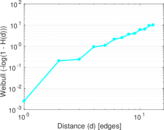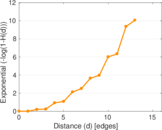### Double Laplacian graph drawing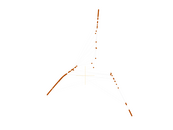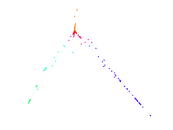### Delaunay graph drawing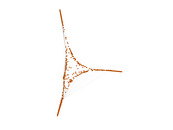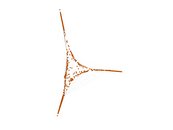### Edge weight/multiplicity distribution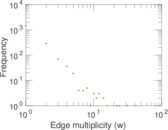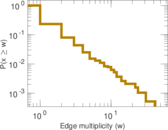### Temporal distribution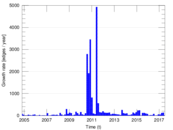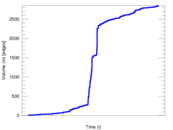### Temporal hop distribution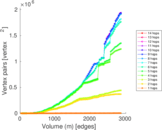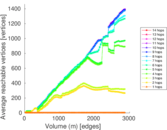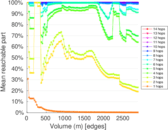### Diameter/density evolution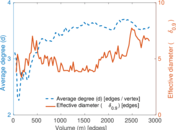### Matrix decompositions plots